## Technical Articles

Tech Articles from your friends at Oracle and the developer community.

# The Impact of Quantum Computing on Software Development

Over the past years and months, interest in quantum computing has increased. On a regular basis, there are new reports from research institutes, companies, or governments about breakthroughs in this field. At the same time, articles with a less technical background talk about the potential consequences of quantum computing, ranging from breaking most of current encryption techniques to a fix for all diseases to complete general AI. Not all expectations are equally realistic, though.

As a realistic, down-to-earth software developer, you may wonder where to draw the line between facts and fiction and how quantum computing will affect software development in the future.

Clearly, we are many years away from production-ready hardware for quantum computing. However, the general principles are clear now, and abstractions allow developers to create applications that leverage quantum computing using simulators.

### Isn't Quantum Computing Just Giving Us More CPU Power?

Traditional software development, using classical computers, translates a high-level programming language (for example, Java) to operations performed on a large number of (hardware) transistors.

The simplified diagram in Figure 1 shows the flow of this process: Java source code is compiled into platform-independent bytecode, which is translated to platform-specific machine code. This allows Java code to work on different operating systems and architectures. The machine code leverages a number of basic operations (gates) acting on memory. The main hardware component to achieve this is the well-known transistor.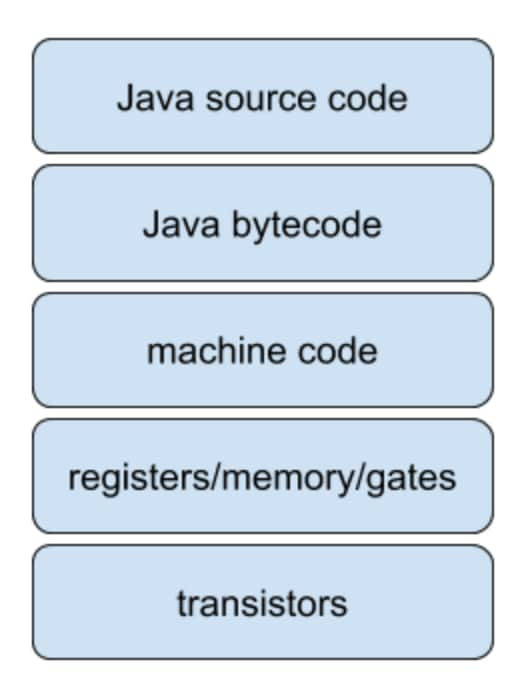Figure 1. Translation of a high-level programming language to operations performed on transistors.

Performance improvements from the past decades have mainly been driven by advantages in hardware technology. The size of a single transistor has been reduced drastically, and more transistors per square millimeter allow for more memory or more processing power.

Quantum computing is disruptive, because it doesn't use a classical transistor as the basic building block; it uses qubits, which we will discuss shortly.

Not only are the basic building blocks different, the gates are different as well. Hence, the stack shown in Figure 1 does not apply to quantum computing.

### Will Quantum Computing Destroy the Whole Stack Up to the Level of Java?

The short answer is "not really." There is a growing consensus among scientists that quantum computers are particularly good for some problems, while classical computers are best for other problems. That should ring a bell: we see the same with GPUs versus CPUs. While GPUs use transistors as well, their operation is different from that of CPUs. However, many applications in a high-level language leverage both a CPU and a GPU under the hood. GPUs are very good for vector processing, and a number of applications and libraries separate work for the CPU from work for the GPU.

This is, for example, the case if you are using JavaFX or Deeplearning4j. If you use JavaFX to create an application that has a user interface, you use Java code only (and maybe FXML to declare the user interface). When the JavaFX scene needs to be rendered on a screen, the JavaFX internal implementations will use shaders and textures and directly talk with the low-level drivers of the GPUs, as shown in Figure 2. As a consequence, you don't need to worry about what part of your code is best suited for the CPU and what part is suited for the GPU.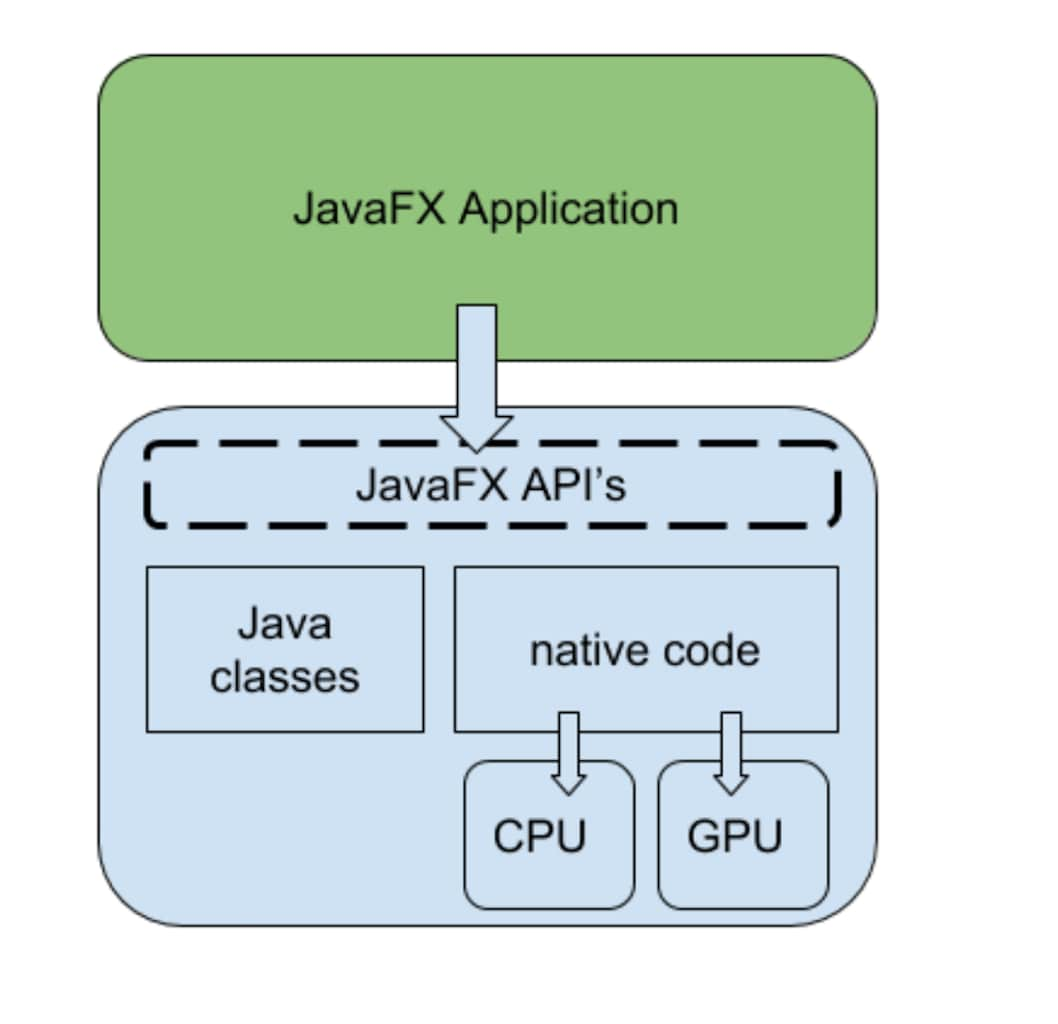Figure 2. JavaFX delegates work to the GPU and the CPU.

As Figure 2 shows, the implementation code for JavaFX delegates work to the GPU and to the CPU. Although this is hidden for the developer (it is not exposed in the API), some knowledge about the GPU often is helpful for creating more-performant JavaFX applications.

If you are using Deeplearning4j, a similar approach is happening. Deeplearning4j has a number of implementations for doing the required vector and matrix operations, and some of those leverage GPUs. As an end developer though, your code does not depend on whether you will be using CPU power or GPU power.

It looks like quantum computers will be excellent in solving problems that typically require resources that scale exponentially with the size of the problem and are, therefore, hard or practically impossible to solve using classical computers. One of the possibilities experts are discussing is a hybrid form of execution: a typical end-to-end application contains classical code that is executed on a CPU, and it can contain quantum code as well.

### How Can a System Execute Quantum Code?

Today, hardware for quantum computers is still extremely experimental. While big companies and presumably some governments are working on prototypes, those are not yet widely available. But even when they will be available, there might be different forms:

• A quantum coprocessor can be integrated with the CPU in a system.
• Quantum tasks can be delegated to quantum cloud systems.

Although there is high uncertainty about the practical implications, there is a growing consensus on how quantum code should look. At the lowest level, this means the basic blocks can be described: a qubit and quantum gates. And as a consequence, quantum simulators can be built that implement the expected behavior.

A quantum simulator is, therefore, a perfect tool to be used during development. The results it produces should be the same as the results on a real hardware quantum computer—but the simulator will be much slower since the quantum effects that speed up quantum hardware have to be simulated using classical software.

### What Are Those Basic Building Blocks for Quantum Computing?

It is often relevant to compare classical computing with quantum computing. In classical computing, we have bits and gates.

A bit can hold a single digit of information, and its value is either 0 or 1. A gate acts upon one or more bits, and it can manipulate those bits. For example, the NOT gate, shown in Figure 3, will flip the value of a bit. If the input is 0, the output of the NOT gate is 1 and vice versa.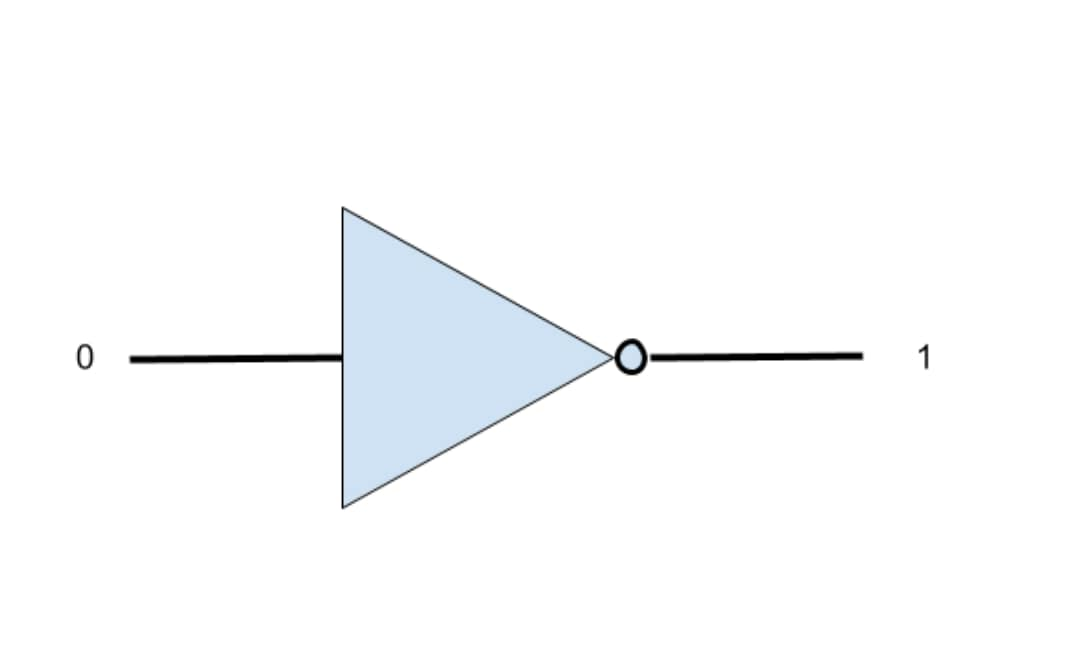Figure 3. NOT gate.

In quantum computing, we have equivalents for bits and gates. The quantum equivalent of a bit is a qubit. A qubit's value can be 0 or 1, similar to a classical bit, but it can also be in a so-called superposition. This is a hard-to-imagine concept that tells us the qubit is in the 0 state and the 1 state at the same time.

When a qubit is in a superposition, its value is a linear combination of the 0 state and the 1 state. We can write this as shown in Figure 4: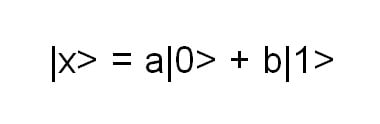Figure 4. Equation for when a qubit is in superposition.

Note that qubits are often written in the bra-ket notation, where the variable name is between a "|" and a ">" symbol.

The expression in Figure 4 tells us that the qubit x is in a superposition of the |0> state and the |1> state. This does not mean that it is in the |0> state OR the |1> state; we don't know its current state.

It is really in both states simultaneously, and it can be manipulated as such. Once we measure the qubit, it will be in a single state though, either |0> or |1>. In the above expression, there is the additional limitation that a^2 + b^2 = 1.

The values of a and b are linked to probabilities: there is an a^2 chance that, when measured, the qubit |x> will contain the value |0>, and there is a b^2 chance that, when measured, the qubit |x> will contain the value |1>.

There is a strong limitation on the joy of quantum computing: Once a qubit is measured, all information about the potential superposition it was in is lost. The qubit will be either 0 or 1.

During calculations, a qubit in a superposition can be both 0 and 1 (with different probabilities). If we have two qubits, those can represent four states (00, 01, 10, and 11), again with different probabilities. This leads to the real power of quantum computers. With eight classical bits, you can represent exactly one number between 0 and 255. All eight bits will be either 0 or 1. With eight qubits, we can represent all numbers between 0 and 255 simultaneously.

### What Is the Benefit of Superposition, If You Can Measure Only a Single State?

In many cases, an algorithm has a simple outcome (for example, "yes" or "no"), but requires lots of parallel computations. By keeping qubits in a superposition during computations, it is possible to take into account all different options at once. Rather than doing evaluations for every single combination, a quantum computer can execute an algorithm on all options in a single step.

An important step in many quantum algorithms is then to link the outcome of the algorithm into a measurement that gives a meaningful result. This is often done leveraging interference: The interesting results are constructively interfering with each other, while the noninteresting results cancel each other by destructive interference.

### How Can a Qubit Be "Transformed" in a Superposition State?

Similar to how classical gates manipulate bits, quantum gates manipulate qubits. Some quantum gates resemble classical gates, for example, the Pauli-X gate brings a qubit from a|0> + b|1> state into b|0| + a|1> state, which is similar to a classical NOT gate. Indeed, when a = 1 and b = 0, the qubit is originally in the |0> state. After applying the Pauli-X gate, this qubit will be in the |1> state, as shown in Figure 5.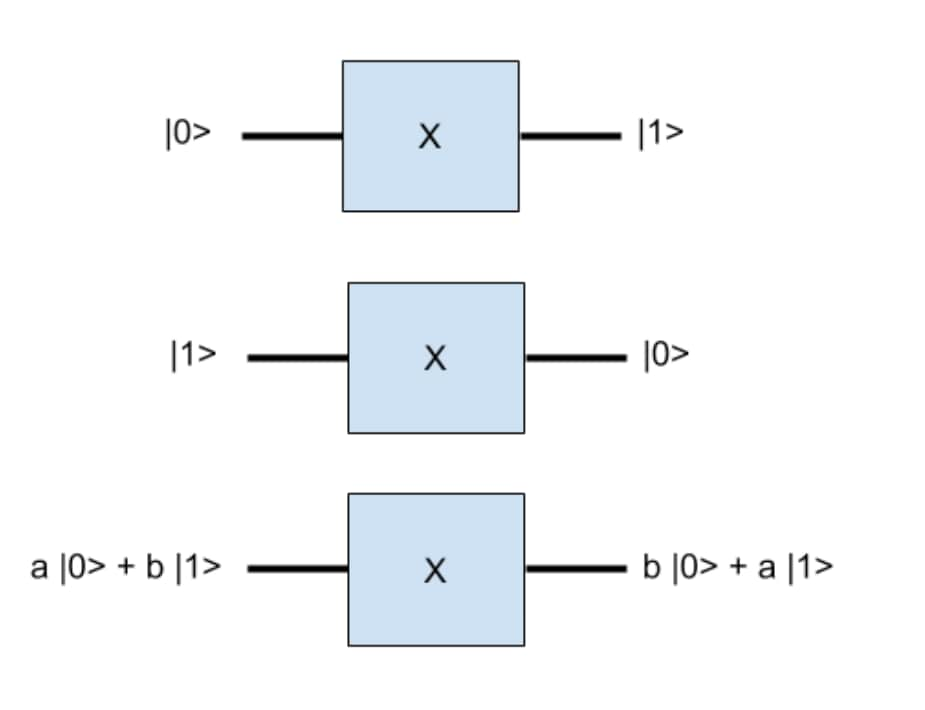Figure 5. Results of applying the Pauli-X gate.

A very interesting gate is the Hadamard gate. This gate will bring a qubit that is in the |0> state into a superposition: 1/sqrt(2)* (|0> + |1>), as shown in Figure 6.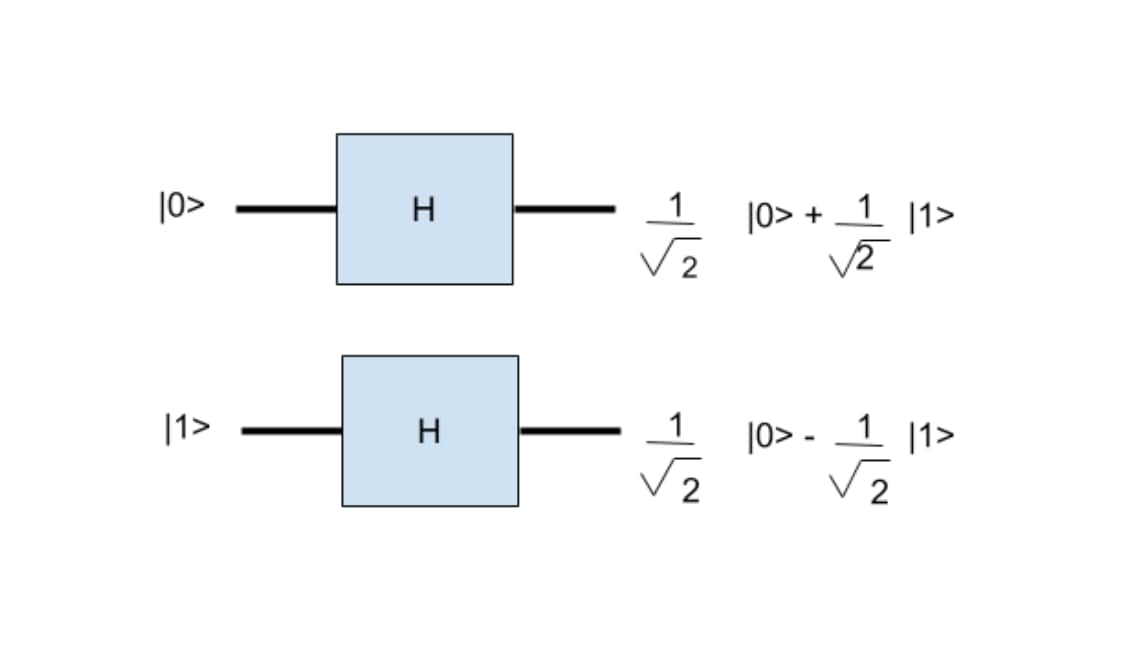Figure 6. Results of applying the Hadamard gate.

After a Hadamard gate is applied to a qubit and the qubit is measured, there is a 50% chance the qubit will have value 0 and a 50% chance it will have value 1. If the qubit is not measured, it stays in a superposition until it is measured.

### How Is All This Possible?

If you really want to know the answer to that question, a deep knowledge of quantum physics is required. But fortunately, you don't need to understand the theory behind this. While the idea of superposition may sound counterintuitive, it should be stressed that this is exactly how the most elementary particles in nature behave. Therefore, quantum computing is much closer to the physical reality of nature than you might think.

### Should I Wait a Few Years to Start Looking into Quantum Computing?

You will be late in the game if you do so. In theory, it is possible to first develop hardware, and then move to the software layer and find out what can be achieved. However, the concepts are more or less clear, which allows quantum simulators to be written in popular languages, including Java, C#, Python, and others.

Those simulators can then be used to work on quantum algorithms. While those algorithms won't have the performance gains that will be obtained when real quantum hardware is used, their functionality should be the same.

Hence, if you work now on your quantum algorithm, you'll have time to create and improve it, and you will be able to run it when the hardware is ready.

Quantum algorithms require a different mindset from classical algorithm. Very smart people started to develop quantum algorithms in the last century, and a growing number of algorithms are published now, including algorithms to factor integers, search lists, deal with path optimization, and more.

There are other reasons why you may want to get involved in quantum computing today. Software systems in large companies typically don't get refactored overnight. However, one of the things that will be shaken by quantum computing is encryption that is based on the theory that it's close to impossible for classical computers to factor large integers into primes.

Although it may take many years before quantum computers are large enough to make integer factorization easily solvable, as software developers we know it also takes many years to change systems and have them using safer technologies.

You can download and use Strange, an open source Java quantum computer simulator. With Strange, you can simulate a quantum algorithm by creating a number of qubits and applying a number of quantum gates to them.

As a very simple example, let's create two qubits, q and q, that initially have the state value 0. We then apply two simple gates to each of the two qubits, which can graphically be represented as shown in Figure 7.

The first qubit will encounter a Pauli-X gate first, followed by a Hadamard gate. The Pauli-X gate will bring it from |0> to |1> and the Hadamard gate will bring it to superposition, with equal probabilities for |0> and |1>. As a consequence, if we execute the circuit 1,000 times, when we measure the first qubit at the end of the circuit, we expect, on average, to find that it has a 0 value 500 times and a 1 value 500 times.

The second qubit is even simpler. We start with an Identity gate, which doesn't change the behavior of the qubit, followed by a Pauli-X gate, which changes its value from 0 to 1.Figure 7. Example of quantum algorithm we can simulate using Strange.

We can create a simple quantum program using Strange that will verify our thinking.

``````

public static void main(String[] args) {
Program p = new Program(2);
Step s = new Step();
Step t = new Step();
SimpleQuantumExecutionEnvironment sqee = new SimpleQuantumExecutionEnvironment();
Result res = sqee.runProgram(p);
Qubit[] qubits = res.getQubits();
Arrays.asList(qubits).forEach(q -> System.out.println("qubit with probability on 1 = "+q.getProbability()+", measured it gives "+ q.measure()));
}

``````

In this application, we create a quantum program with two qubits:

``````

Program p = new Program(2);

``````

We apply two steps in this program. In the first step, we apply an Pauli-X gate to q. We don't apply a gate to q, which implicitly means we apply the Identity gate to it. We add this step to the program:

``````

Step s = new Step();

``````

We then create the second step, in which we apply a Hadamard gate to q and a Pauli-X gate to q, and we add that step to the program:

``````

Step t = new Step();

``````

Now that our program is ready, we want to execute it. Strange comes with a built-in quantum simulator, but it can also use a cloud service to execute programs in a cloud environment (for example, on Oracle Cloud).

In the following sample, we use the simple built-in simulator, run the program, and obtain the resulting qubits:

``````

SimpleQuantumExecutionEnvironment sqee = new SimpleQuantumExecutionEnvironment();
Result res = sqee.runProgram(p);
Qubit[] qubits = res.getQubits();

``````

Before we measure the qubits (and lose all information), we print the probabilities. Next, we measure the qubits and print the value:

``````

Arrays.asList(qubits).forEach(q -> System.out.println("qubit with probability on 1 = "+q.getProbability()+", measured it gives "+ q.measure()));

``````

If you run this application, you'll see the following output:

``````

qubit with probability on 1 = 0.50, measured it gives 1
qubit with probability on 1 = 1, measured it gives 1

``````

Note that the measured value for the first qubit can be 0 as well, as we expected.

If you run this multiple times, the measured value of the first qubit will give, on average, the same amount of 0s as 1s.

### So Is That All There Is to Know About Quantum Computing?

Far from it. We didn't touch a number of important concepts such as entanglement, which allows for interactions between qubits even if they are physically far away from each other. We didn't discuss any of the known quantum algorithms, including Shor's algorithm that allows factoring integers into primes. Also, we ignored a number of mathematical and physical facts, for example, the fact that in a superposition |x> = a|0> + b|1>, both a and b can be complex numbers.

Johan Vos, ( @johanvos) started to work with Java in 1995. He was part of the Blackdown team, porting Java to Linux. His main focus is on end-to-end Java, combining back-end systems and mobile/embedded devices. He received a Duke's Choice Award in 2014 for his work on JavaFX on mobile. In 2015, he cofounded Gluon, which allows enterprises to create mobile Java client applications leveraging existing back-end infrastructure. Gluon received a Duke's Choice Award in 2015. He is the colead of OpenJFX and the project lead of OpenJDK Mobile. Vos is a Java Champion, a member of the Belgian Java User Group (BeJUG) and Devoxx steering groups, and he is a JCP member. He is the lead author of the Pro JavaFX 8 book, and he has been a speaker at numerous conferences on Java.

Latest content

Explore and discover our latest tutorials

## Serverless functions

Serverless functions are part of an evolution in cloud computing that has helped free organizations from many of the constraints of managing infrastructure and resources.

## What is a blockchain?

In broad terms, a blockchain is an immutable transaction ledger, maintained within a distributed peer-to-peer (p2p) network of nodes. In essence, blockchains serve as a decentralized way to store information.

## OCI CLI

The CLI is a small-footprint tool that you can use on its own or with the Console to complete Oracle Cloud Infrastructure tasks. The CLI provides the same core functionality as the Console, plus additional commands.